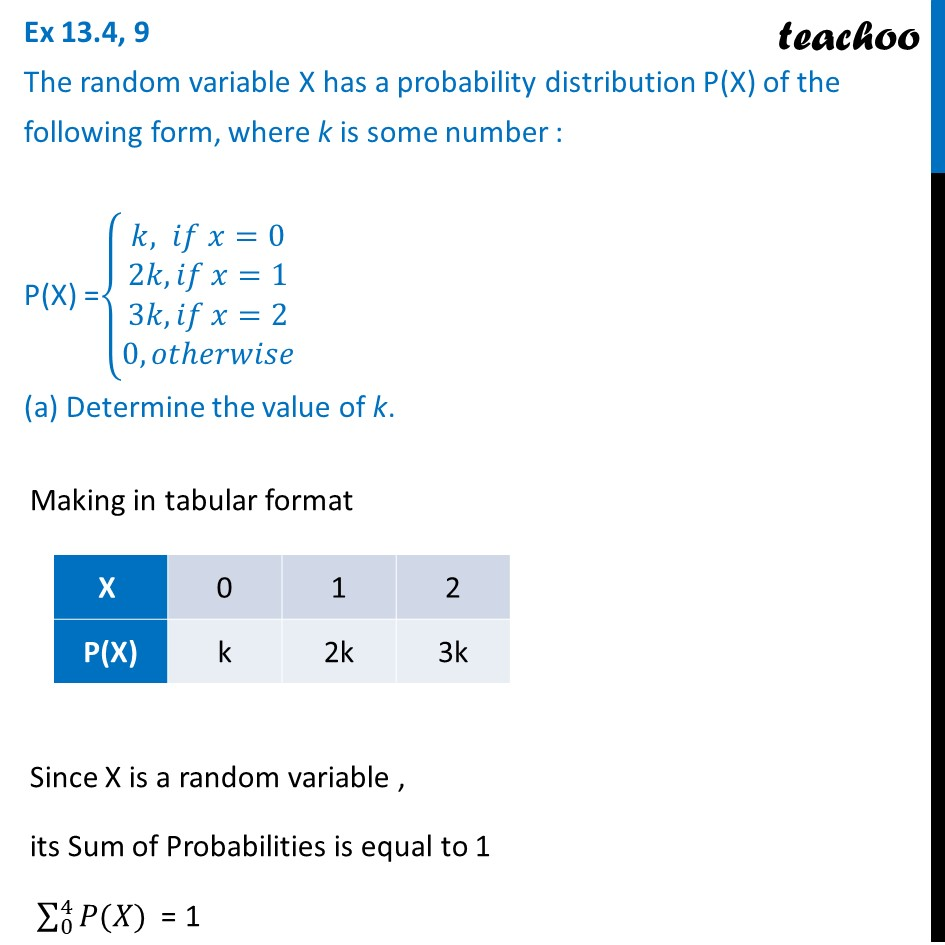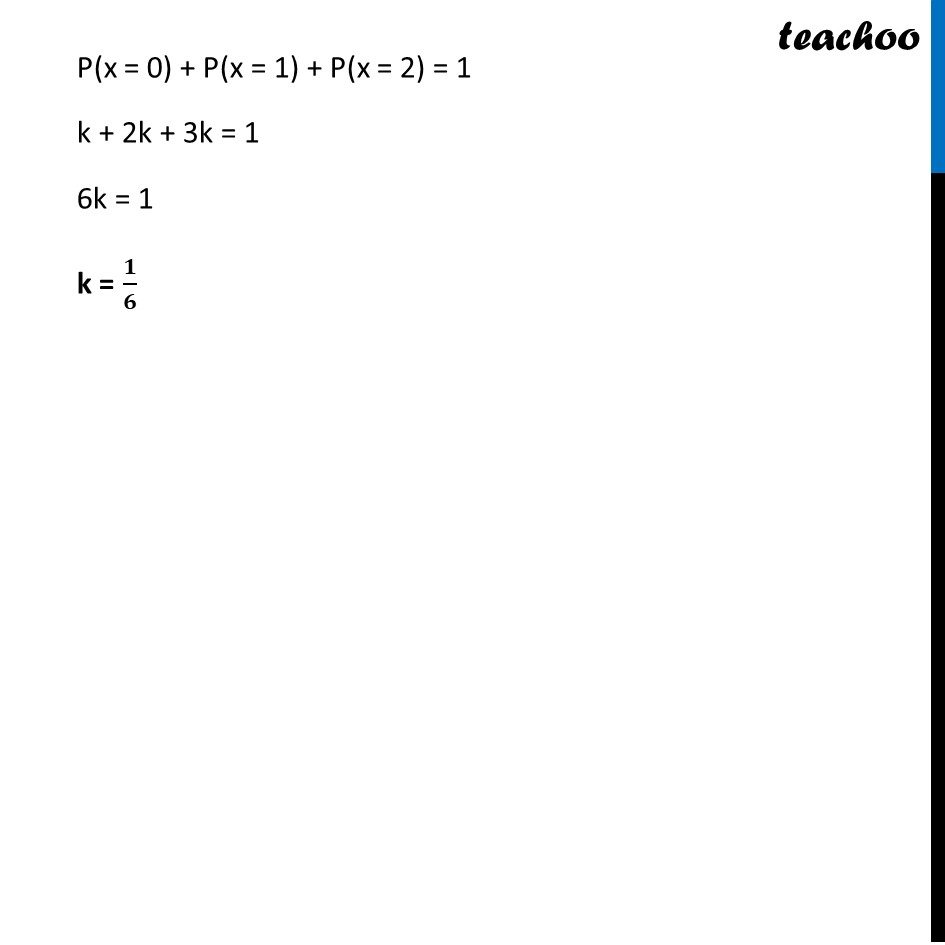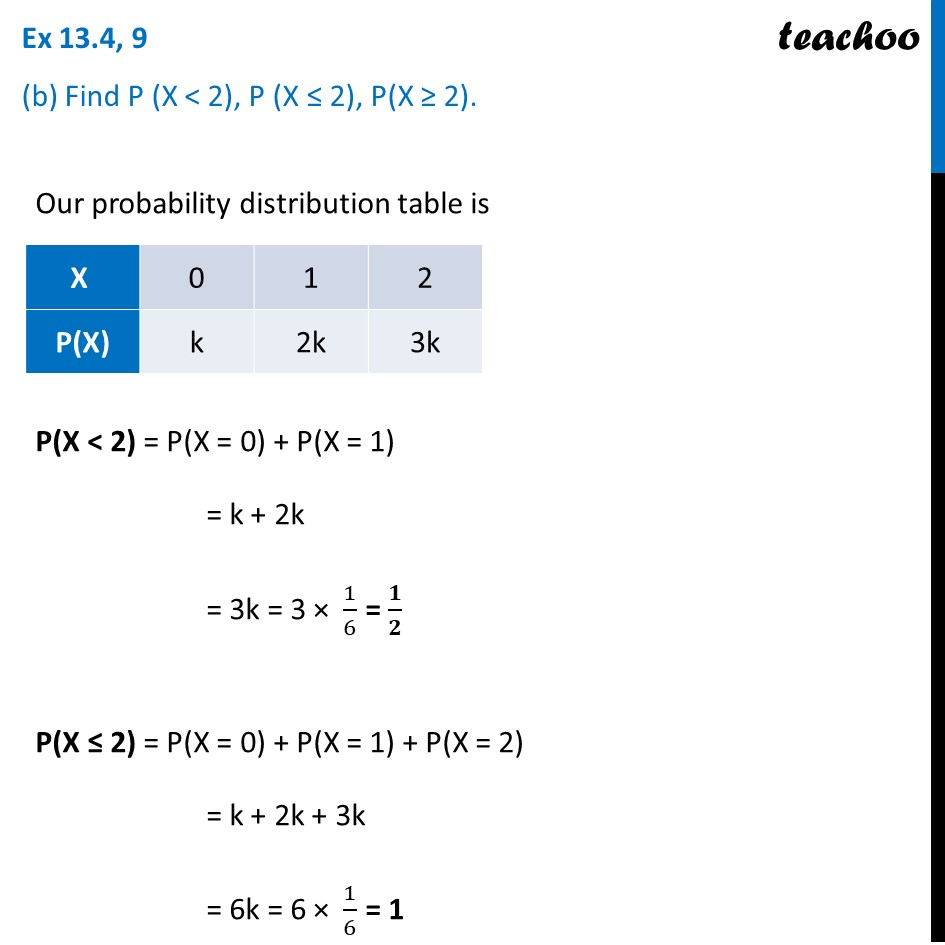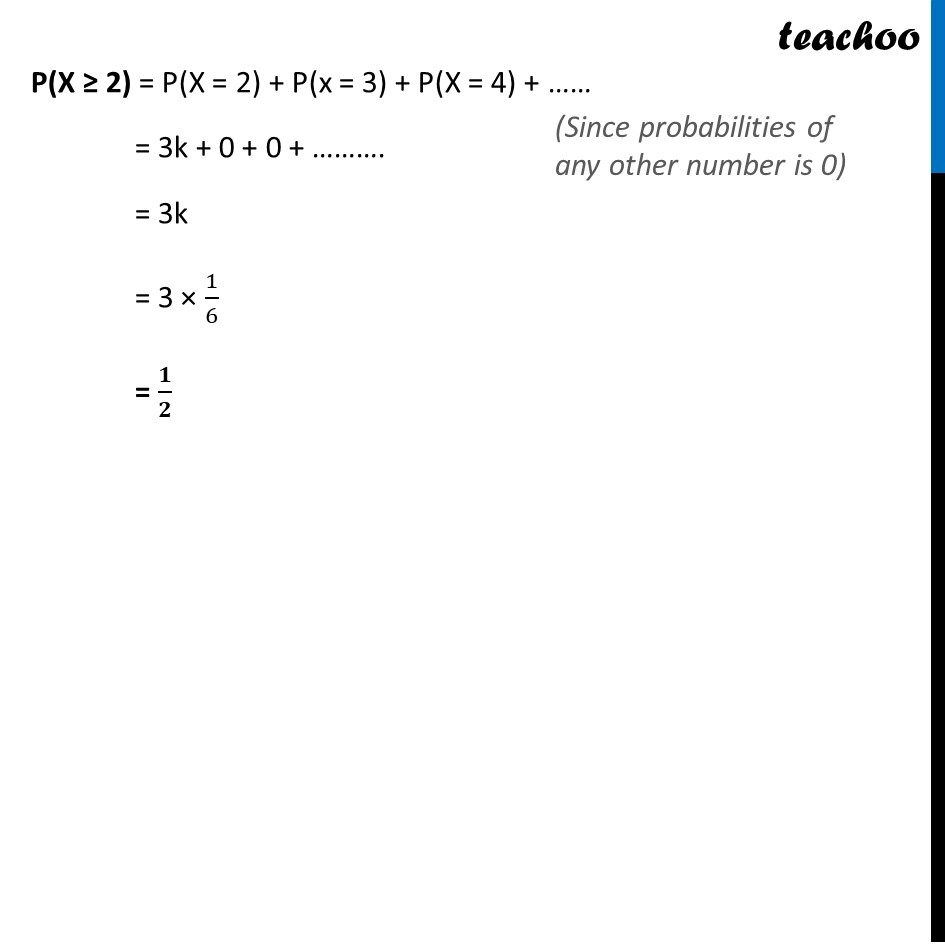Probability Distribution

Chapter 13 Class 12 Probability
Serial order wiseLearn in your speed, with individual attention - Teachoo Maths 1-on-1 Class

### Transcript

Question 9 The random variable X has a probability distribution P(X) of the following form, where k is some number : P(X) = 𝑘, 𝑖𝑓 𝑥=0﷮2𝑘, 𝑖𝑓 𝑥=1﷮3𝑘, 𝑖𝑓 𝑥=2﷮0, 𝑜𝑡ℎ𝑒𝑟𝑤𝑖𝑠𝑒﷯﷯ (a) Determine the value of k. Making in tabular format Since X is a random variable , its Sum of Probabilities is equal to 1 0﷮4﷮𝑃(𝑋)﷯ = 1 P(x = 0) + P(x = 1) + P(x = 2) = 1 k + 2k + 3k = 1 6k = 1 k = 𝟏﷮𝟔﷯ Question 9 (b) Find P (X < 2), P (X ≤ 2), P(X ≥ 2). Our probability distribution table is P(X < 2) = P(X = 0) + P(X = 1) = k + 2k = 3k = 3 × 1﷮6﷯ = 𝟏﷮𝟐﷯ P(X ≤ 2) = P(X = 0) + P(X = 1) + P(X = 2) = k + 2k + 3k = 6k = 6 × 1﷮6﷯ = 1 P(X ≥ 2) = P(X = 2) + P(x = 3) + P(X = 4) + …… = 3k + 0 + 0 + ………. = 3k = 3 × 1﷮6﷯ = 𝟏﷮𝟐﷯